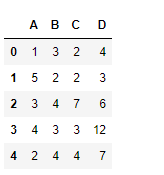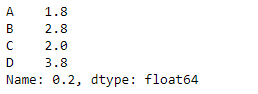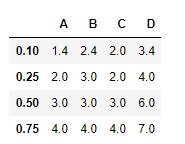GFG App
Open AppBrowser
Continue

# Python | Pandas dataframe.quantile()

Python is a great language for doing data analysis, primarily because of the fantastic ecosystem of data-centric python packages. Pandas is one of those packages and makes importing and analyzing data much easier. Pandas dataframe.quantile() function return values at the given quantile over requested axis, a numpy.percentile. Note : In each of any set of values of a variate which divide a frequency distribution into equal groups, each containing the same fraction of the total population.

Syntax: DataFrame.quantile(q=0.5, axis=0, numeric_only=True, interpolation=’linear’)

Parameters : q : float or array-like, default 0.5 (50% quantile). 0 <= q <= 1, the quantile(s) to compute

axis : [{0, 1, ‘index’, ‘columns’} (default 0)] 0 or ‘index’ for row-wise, 1 or ‘columns’ for column-wise

numeric_only : If False, the quantile of datetime and timedelta data will be computed as well

interpolation : {‘linear’, ‘lower’, ‘higher’, ‘midpoint’, ‘nearest’}

Returns : quantiles : Series or DataFrame -> If q is an array, a DataFrame will be returned where the index is q, the columns are the columns of self, and the values are the quantiles. -> If q is a float, a Series will be returned where the index is the columns of self and the values are the quantiles.

Example #1: Use quantile() function to find the value of “.2” quantile

## Python3

 `# importing pandas as pd` `import` `pandas as pd`   `# Creating the dataframe ` `df ``=` `pd.DataFrame({"A":[``1``, ``5``, ``3``, ``4``, ``2``],` `                   ``"B":[``3``, ``2``, ``4``, ``3``, ``4``],` `                   ``"C":[``2``, ``2``, ``7``, ``3``, ``4``], ` `                   ``"D":[``4``, ``3``, ``6``, ``12``, ``7``]})`   `# Print the dataframe` `df`Let’s use the dataframe.quantile() function to find the quantile of ‘.2’ for each column in the dataframe

## Python3

 `# find the product over the index axis` `df.quantile(.``2``, axis ``=` `0``)`

Output :Example #2: Use quantile() function to find the (.1, .25, .5, .75) quantiles along the index axis.

## Python3

 `# importing pandas as pd` `import` `pandas as pd`   `# Creating the dataframe ` `df ``=` `pd.DataFrame({"A":[``1``, ``5``, ``3``, ``4``, ``2``],` `                   ``"B":[``3``, ``2``, ``4``, ``3``, ``4``],` `                   ``"C":[``2``, ``2``, ``7``, ``3``, ``4``],` `                   ``"D":[``4``, ``3``, ``6``, ``12``, ``7``]})`   `# using quantile() function to` `# find the quantiles over the index axis` `df.quantile([.``1``, .``25``, .``5``, .``75``], axis ``=` `0``)`

Output :My Personal Notes arrow_drop_up